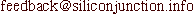# Using Transition Curves

While designing my new N scale dog-bone layout, I encountered the need to use transition curves. The minimum radius on this layout is 280 mm(11") which is on the lower side. This makes the use of transition curves more important. Apart from simple transition curves designed to ease the transition from a straight to a curve, this layout had a more complicated transition. This led me to attempt to analyze different situations where one would use transition curves. Let us look at a few situations involving of transition curves.

A transition curve can be seen as a curve where the radius at a point on the curve changes gradually as you move along the curve. Let us adopt a convention of using a radius of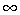for a straight line, r for a curve and -r when the centre for the radius is on the other side of the curve. Some situations where one or more transition curves are needed are described below. I have not done any simulations or actual operation using the suggested curves.

## Straight to Curve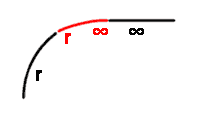This is the most common example where a transition curve is used. The straight is a tangent to the curve. A transition curve with the radius from the straight going fromto r where r is the radius of the curve is used.

## Curve to Curve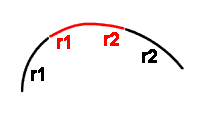This assumes that both curves have their centres on the same side. A transition curve with the radius going from r1 to r2 where r1 and r2 are the radii of the two curves is used.

## Curve to Reverse Curve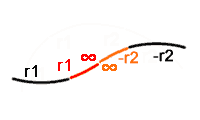This is the S curve commonly encountered on many layouts. Two transition curves are needed. The first starts from the curve with a radius of r1 transitioning from a radius of r1 to. This is followed by a second transition curve going fromto -r2. r1 and r2 are the radii of the two curves.

## Straight to Straight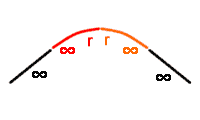The two straights are at an angle. Two transition curves are needed. The first goes fromto a radius r and the second is a mirror image with the transition going from r to. The radius r is an arbitrary radius. It should be greater than or equal to the minimum radius for the layout. A small value for r will result a short, sharp curve between the straights.

## Offset Straight to Curve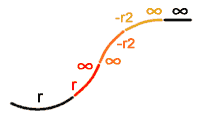This is the most complicated situation I came across. Unfortunately, this was the situation I encountered in my simple dog-bone layout. The situation is that of a straight that enters a curve at an angle rather than at a tangent to the curve. This requires three consecutive transition curves. The first goes from r towhere r is the radius of the curve. This will result in a transition that ends in a straight line except that it is at an angle to the offset straight. This is the same as the situation described in the previous example - that of two straights at an angle. We have seen that this can be solved by having two transition curves. This gives us the solution involving three transition curves.

The last situation is what I encountered in my dog-bone layout. I designed the profile using an Excel spreadsheet. I eye-balled the resulting curve using the graph facility in Excel. Once satisfied, I converted the coordinates in the Excel spreadsheet to a series of macro instructions for my CAD package. Running the macro drew the resulting transition curves straight into the drawing of my layout. Unfortunately, it extended beyond the edge of the lobes of plywood which I had already cut in advance. Several iterations along with the reducing of the minimum radius to 250 mm(10") gave me a curve that fitted within the plywood base. The lower minimum radius will hopefully be tolerable with the use of transition curves. The resulting curve was then printed in full scale on several pages which were mosaiced together using sticky tape. This was then transferred on to the plywood base and the line was used to guide the laying of the track. The line for the edge of the ballast strip was drawn rather than the centre of the track. This simplified laying the track and the ballast. The ballast strip is fairly uniform in width.

A transition curve is a curve where the curvature(reciprocal of the radius) changes linearly as you move along the curve. This curve is called a clothoid. A simplified version of this curve is the cubic spiral where the curvature changes linearly as you move along the X axis. this works well and is a reasonable approximation for short curves. All transitions used on my layout are cubic spirals. Due to the additional complexity of going through several iterations of arranging the transition curves, the approach of using a spreadsheet to calculate the extent of the curves was used. In more mundane use of transition curves, I use my Cubic Spiral Template.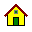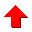Updated on 5 Dec 2000. Feedback to